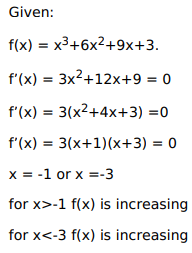# Solve this following

Question:

Mark $(\sqrt{)}$ against the correct answer in the following:

The function $f(x)=x^{3}+6 x^{2}+9 x+3$ is decreasing for

A. $1 B.$x>1$C.$x<1$D.$x<1$or$x>3$Solution:But for$-1